Download PDF文章快速检索 高级检索

Statistical evaluation of the clustering results based on permutation test
GU Feiyang, TIAN Bo, ZHANG Simeng, CHEN Zheng, HE ZengyouSoftware School, Dalian University of Technology, Dalian 116621, China
Abstract: For the result of clustering, tranditional methods of evalution couldn't assess the result in statistics. We propose a new algorithm called ECP(Statistical evaluation of Clustering based on Permutation test) which uses permutation test to evaluate the result of clustering. To evaluate the performance of the algorithm, we use the data sets, iris, wine, yeast, from UCI datasets. Experimental results show that the performance of the algorithm is good.
Key words: clustering     clustering evaluation     statistical test     permutation test

1 相关研究 1.1 利用簇结构评估聚类质量

1.2 SigClust

SigClust认为如果一个数据集符合高斯分布，那么对这个数据集的任何分割都是不合理的。因此这个方法的前提假设是：一个单一的簇的元素符合高斯分布。SigClust主要是针对k=2的聚类评估。对于k>2的情况，还没有比较好的解决办法。

1.3 层次聚类的p-value计算

2 基本概念 2.1 无监督聚类质量评估函数

 ${\text{DBI}} = \frac{1}{k}\sum\limits_{i = 1}^k {\max \left( {\frac{{{S_i} + {S_j}}}{{{D_{ij}}}}} \right)} .$

DBI的思想是一个高质量的聚类结果需要满足:同一个簇的各元素间相似度大，不同类之间的相似度小。在DBI中，分子越小意味着簇内元素相似度越大，分母越大意味着簇间相似度越小。

2.2 聚类评估的p-value

 ${P_{perm}} = \frac{{\sum\nolimits_{n = 1}^{{N_{{\text{all}}}}} {I\left( {{x_n} ≤ {x_0}} \right)} }}{{{N_{{\text{all}}}}}}$

 ${P_{perm0}} = \frac{{\sum\nolimits_{n = 1}^N {I\left( {{x_n} ≤ {x_0}} \right)} }}{N}.$

 ${P_{perm1}} = \frac{{1 + \sum\nolimits_{n = 1}^N {1\left( {{x_n} ≤ {x_0}} \right)} }}{{N + 1}}$

3 基于置换检验的聚类结果评估 3.1 基本思想

for i← 0 to n-1 do

index ← rand() mod (i + 1)

swap(CIi, CIindexCIi, CIindex)

for i ← 1 to N do

Shuffle(CI, n)

3.2 加速技巧

for i← 1 to N do

 ${\text{DBI}} = \frac{1}{k}\sum\limits_{i = 1}^k {\max \left( {\frac{{{S_i} + {S_j}}}{{{D_{ij}}}}} \right)} .$
Si的定义可以得出:
 ${S_i} = \sqrt {\frac{{\sum\nolimits_{j = 1}^{{m_i}} {{{\left\| {{z_j} - \bar z} \right\|}^2}} }}{{{m_i}}}} .$

 $\sum\nolimits_{j = 1}^{{m_i}} {{{\left\| {{z_j} - \bar z} \right\|}^2}} = \sum\nolimits_{t = 1}^d {\sum\nolimits_{j = 1}^{{m_i}} {{{\left( {{a_{jt}} - {{\bar a}_t}} \right)}^2}} } ,$

 $\begin{gathered} \frac{{\sum\nolimits_{j = 1}^{{m_i}} {{{\left\| {{z_j} - \bar z} \right\|}^2}} }}{{{m_i}}} = \frac{{\sum\nolimits_{t = 1}^d {\sum\nolimits_{j = 1}^{{m_i}} {{{\left( {{a_{jt}} - {{\bar a}_t}} \right)}^2}} } }}{{{m_i}}} = \hfill \\ \sum\nolimits_{t = 1}^d {\frac{{\sum\nolimits_{j = 1}^{{m_i}} {{{\left( {{a_{jt}} - {{\bar a}_t}} \right)}^2}} }}{{{m_i}}}} \hfill \\ \end{gathered}$

 $\frac{{\sum\nolimits_{j = 1}^{{m_i}} {{{\left( {{a_{jt}} - {{\bar a}_t}} \right)}^2}} }}{{{m_i}}} = \frac{{\sum\nolimits_{j = 1}^{{m_i}} {{a_{jt}}^2} }}{{{m_i}}} - {{\bar a}_t}^2$

 $\frac{{\sum\nolimits_{j = 1}^{{m_i}} {{{\left\| {{z_j} - \bar z} \right\|}^2}} }}{{{m_i}}} = \sum\nolimits_{t = 1}^d {\frac{{\sum\nolimits_{j = 1}^{{m_i}} {{a_{jt}}^2} }}{{{m_i}}}} - {{\bar a}_t}^2$

${\sum\nolimits_{j = 1}^{{m_i}} {{a_{jt}}^2} }$是簇i中所有元素中第t维的平方和，${{\bar a}_t}$是簇i中所有元素第t维的平均值。所以为了计算Si，每一维只需要维护两个值就可以了:平方和与平均值。当簇标号交换的话，能在O(1)复杂度内修正这两个值。修改完每个维度的这两个值后，就可以用DB-Index算出函数值了。

3.3 更准确的p-value

 $\mu = \frac{{\sum\nolimits_{i = 1}^N {{x_i}} }}{N}$ (1)
 $\partial = \sqrt {\frac{{{{\left( {{x_i} - \bar x} \right)}^2}}}{{N - 1}}}$ (2)

4 实验

4.1 利用p-value选择合适的聚类算法

 数据 算法 p-value f-score accuracy Iris Random 0.456 254 1.134 140 0.380 000 Hierarchical Clustering 0.100 548 1.656 570 0.666 667 DBSCAN 0.042 825 2.714 400 0.906 667 k-means 0.042 751 2.655 840 0.886 667 Wine Random 0.559 588 1.095 420 0.410 112 Hierarchical Clustering 0.001 574 1.666 460 0.657 303 DBSCAN 1.892 991e-05 2.833 750 0.943 820 k-means 1.818 384e-05 2.832 200 0.943 820 Yeast Random 0.688 145 1.078 260 0.357 198 Hierarchical Clustering 0.003 871 0.835 371 0.360 277 DBSCAN 0.000 711 1.304 800 0.434 950 k-means 7.544 556 e-05 1.881 950 0.480 370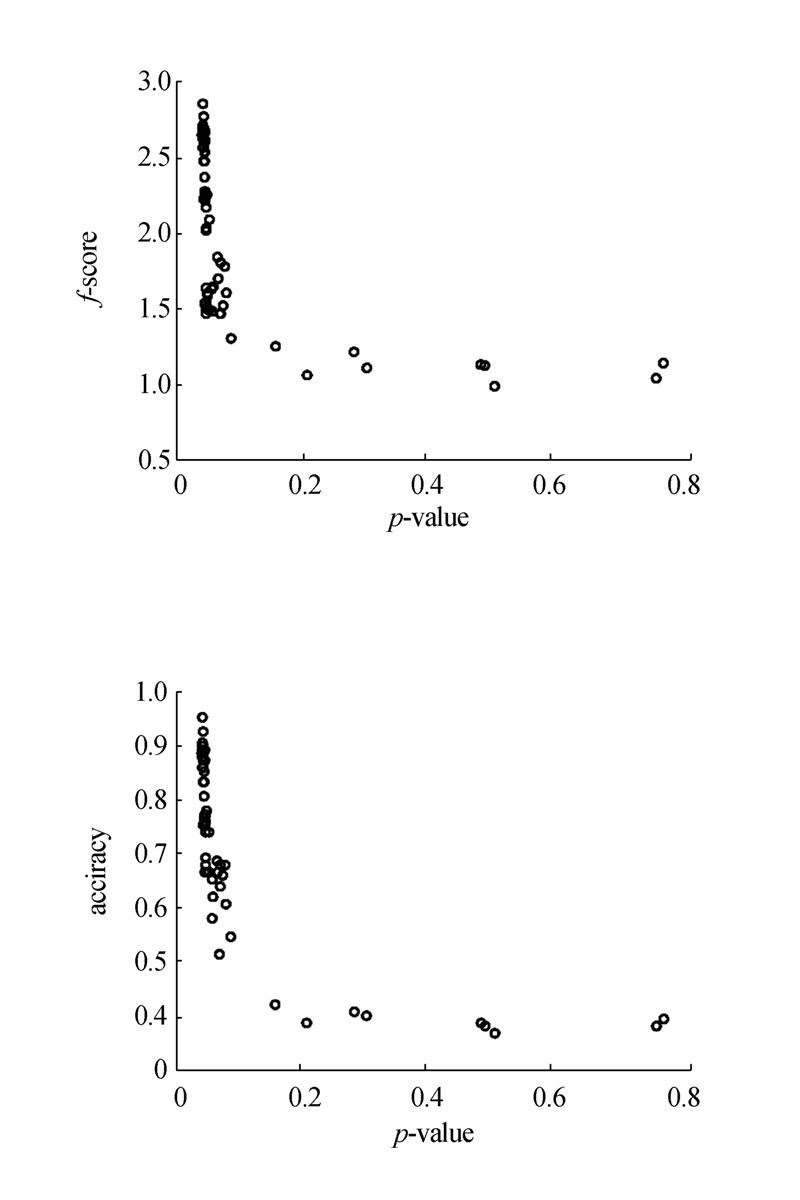图 1 Iris数据集p-value与f-score和accuracy的关系Fig. 1 The relationship between p-value and f-score, accuracy of iris dataset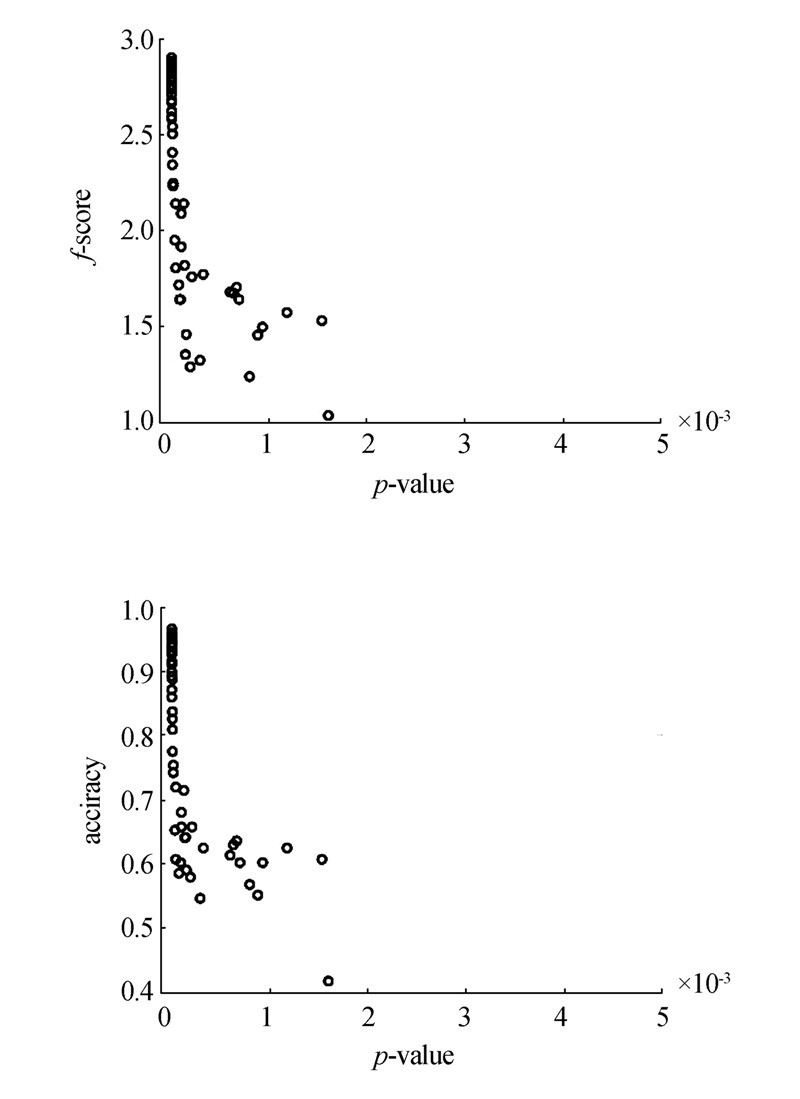图 2 Wine数据集p-value与f-score和accuracy的关系Fig. 2 The relationship between p-value and f-score, accuracy of wine dataset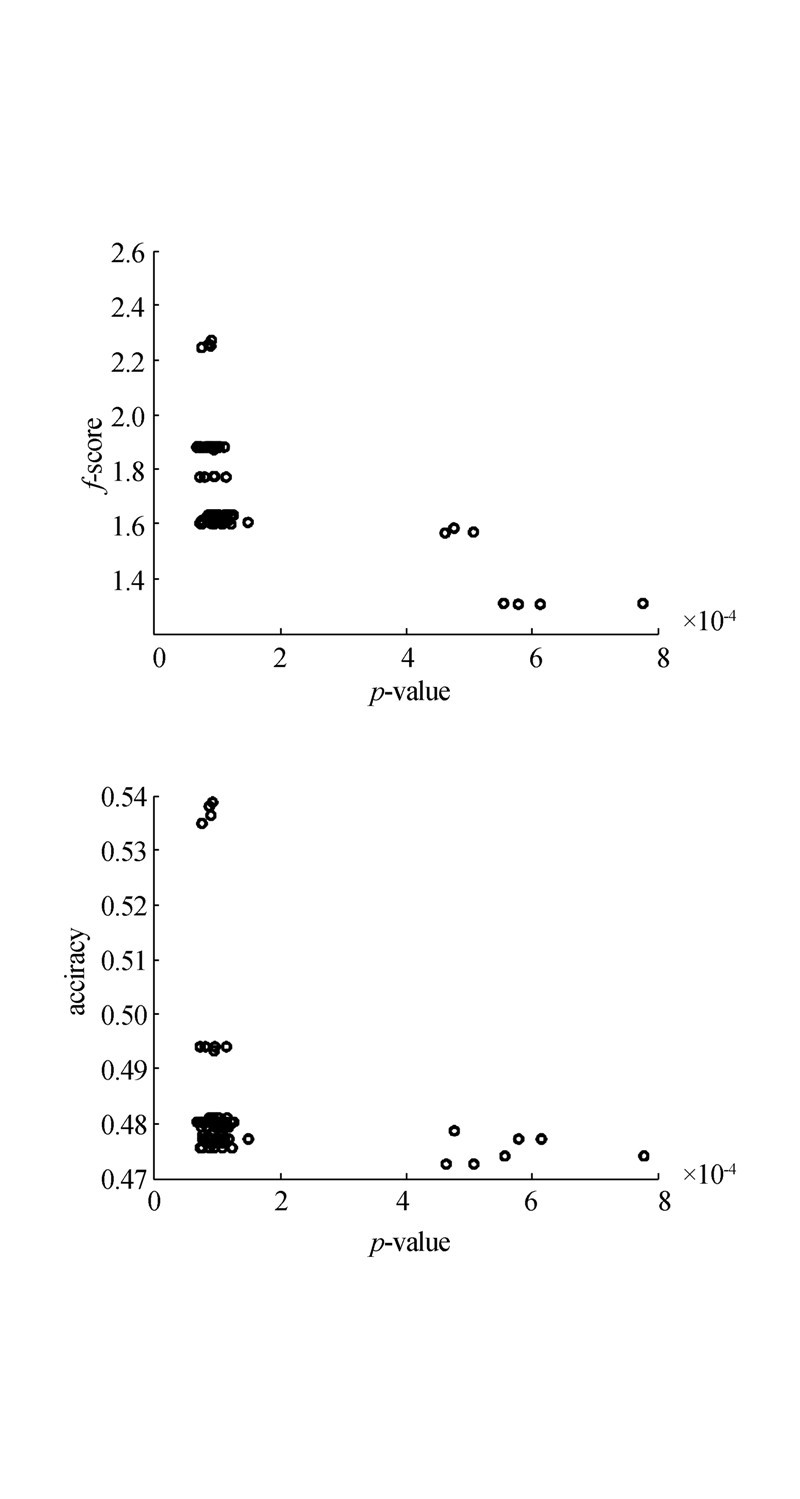图 3 Yeast数据集p-value与f-score和accuracy的关系Fig. 3 The relationship between p-value and f-score, accuracy of yeast dataset
4.2 利用p-value决定数据集簇的数目k

 $R\left( i \right) = \frac{{p\left( {i - 1} \right)}}{{p\left( i \right)}},$

 数据 2 3 4 5 6 7 Iris 0.108 518 0.042 742 0.020 435 0.017 261 0.006 991 0.003 208 Wine 0.001 946 1.988 773e-05 7.579 904e-07 2.381 891e-08 2.125 773e-09 1.537 855e-09 Yeast 0.006 911 0.001 040 6.937 873e-05 9.647 412e-06 1.327 582e-06 3.264 579e-06

 数据 3 4 5 6 7 Iris 2.538 900 2.091 640 1.183 870 2.469 150 2.179 010 Wine 97.836 510 26.237 440 31.823 050 11.204 820 1.382 300 Yeast 6.644 860 14.991 890 7.191 430 7.266 900 0.406 660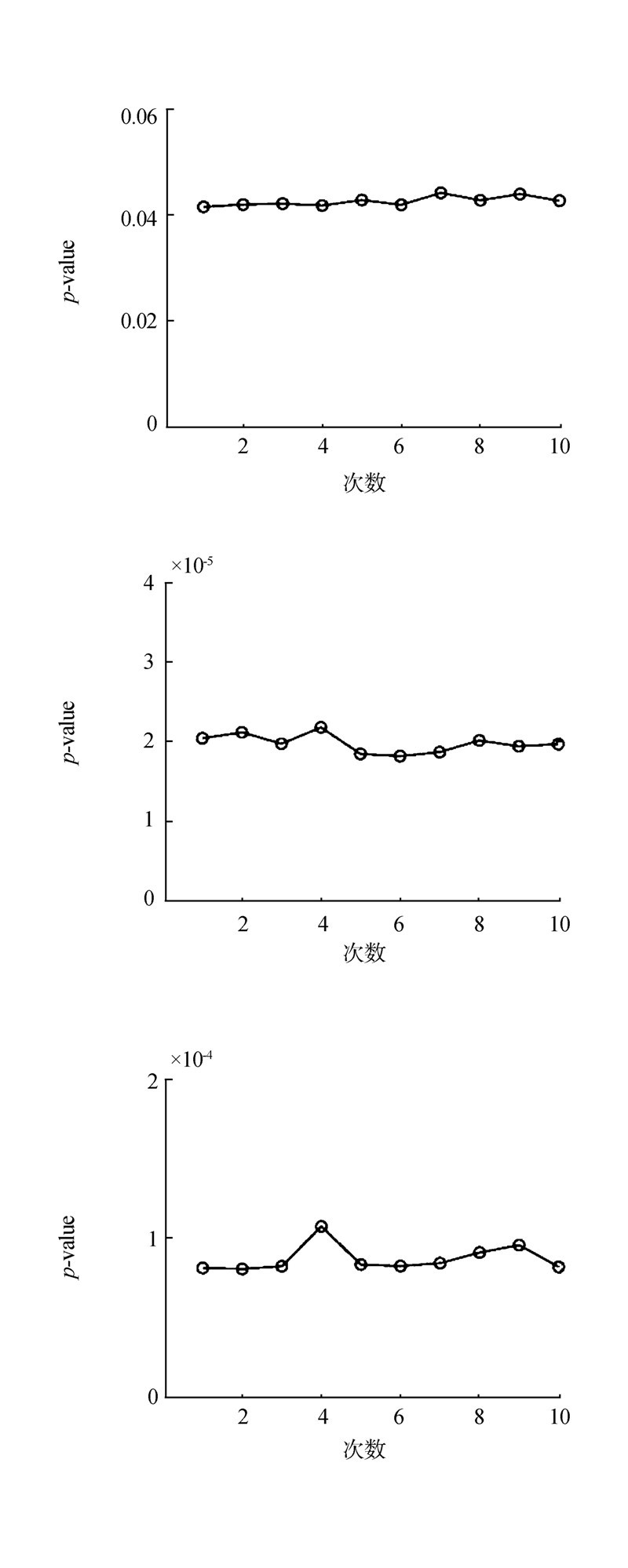图 4 p-value 稳定性Fig. 4 The stability of p-value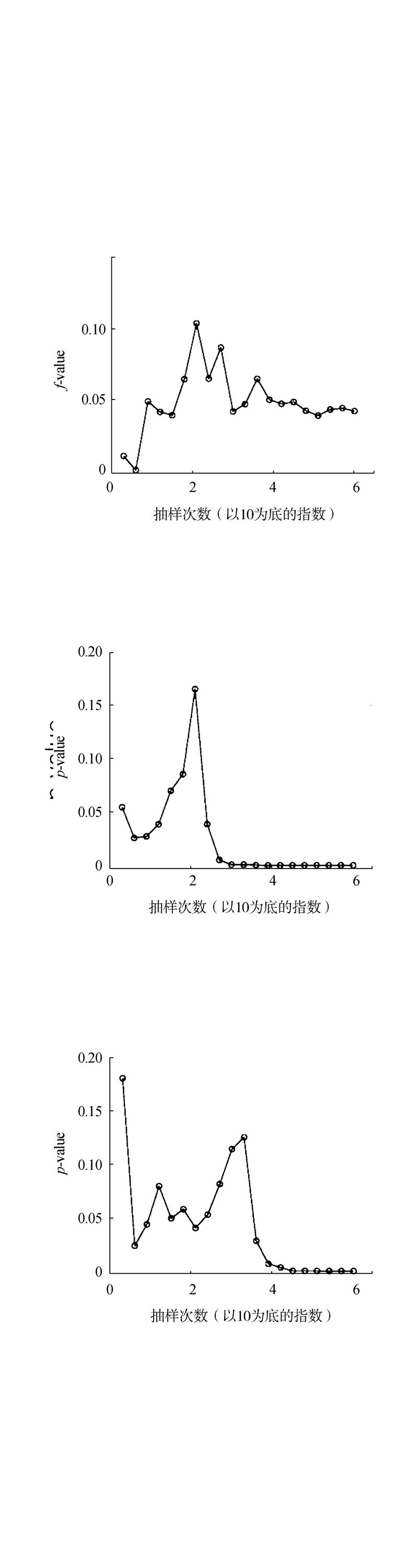图 5 p-value与抽样次数的关系Fig. 5 The relationship between p-value and the number of samples

4.3 与相关算法对比 4.3.1 ECP与最大熵模型比较

4.3.2 ECP与SigClust对比

SigClust算法是主要针对k为2聚类结果的评估。本文从每个数据集中选出了两类用k-means进行聚类(比如iris数据集中选出了Setosa、Versicolour两类进行对比)。为了让聚类质量有层次的差距，对k-means的聚类结果进行不同程度的破坏。破坏的程度越大，聚类的质量越差。实验结果如表 5。从实验看SigClust与ECP都能够区别出很好和很差的聚类。但是可以很明显地看出，SigClust对聚类质量的区分度不够大。比如对于iris数据集计算的f1为2和1.8，SigClust算出的p-value都是0，没有区分开这2个不同划分的质量。同样地iris数据集f1为1.36和1.158 65，SigClust算出的p-value都为1。实验可以看出ECP能很好地区分聚类质量的差距。因此，与SigClust相比，ECP不仅能处理k>2的情况，而且能更好地评估聚类质量。

 算法 iris wine yeast 最大熵 0.001 0.001 0.001 最大熵 拟合正态 4.891 817e-05 0.370 035 2 0.002 626 655 ECP 0.042 742 13 1.988 773e-05 6.937 873e-05

 数据 p-value/ECP p-value/Sigclust Sigclust f-score accuracy Iris 0.114 572 8 0 2 1 0.121 688 1 0 1.8 0.9 0.157 168 9 1 1.36 0.68 0.228 296 5 1 1.158 65 0.58 wine 0.001 534 783 0 1.876 81 0.938 462 0.002 878 496 0.199 2 1.673 66 0.838 462 0.006 082 356 1 1.430 74 0.715 385 0.221 656 1 1.011 64 0.546 154 yeast 0.006 761 993 0 1.130 05 0.567 265 0.010 775 1 1 1.077 86 0.539 238 0.012 549 87 1 1.073 48 0.536 996 0.256 406 2 1 1.044 03 0.522 422
4.3.3 ECP与ECP1对比

 算法 iris wine yeast ECP1 18 s 50 s 56s ECP 1109 s 734 s 280 m
5 结束语

  TAN Pangning, STEINBACH M, KUMAR V. Introduction to data mining[M]. Boston: Addison-Wesley, 2005.  HAN Jiawei, KAMBER M, PEI Jian. Data mining: concepts and techniques[M]. 3rd ed. Burlington, MA, USA: Elsevier, 2012: 1-33.  尹宏伟, 李凡长. 谱机器学习研究综述[J]. 计算机科学与探索, 2015, 9(12): 1409-1419. YIN Hongwei, LI Fanzhang. Survey on spectral machine learning[J]. Journal of frontiers of computer science and technology, 2015, 9(12): 1409-1419.  JAIN A K, MURTY M N, FLYNN P J. Data clustering: a review[J]. ACM computing surveys, 1999, 31(3): 264-323.  WU Xindong, KUMAR V, QUINLAN J R, et al. Top 10 algorithms in data mining[J]. Knowledge and information systems, 2008, 14(1): 1-37.  HALKIDI M, BATISTAKIS Y, VAZIRGIANNIS M. On clustering validation techniques[J]. Journal of intelligent information systems, 2001, 17(2-3): 107-145.  HANDL J, KNOWLES J, KELL D B. Computational cluster validation in post-genomic data analysis[J]. Bioinformatics, 2005, 21(15): 3201-3212.  KONTONASIOS K N, VREEKEN J, DE BIE T. Maximum entropy modelling for assessing results on real-valued data[C]//Proceedings of the 11th international conference on data mining. Vancouver, BC, Canada, 2011: 350-359.  OJALA M. Assessing data mining results on matrices with randomization[C]//Proceedings of international conference on data mining. Sydney, Australia, 2010: 959-964.  OJALA M, VUOKKO N, KALLIO A, et al. Randomization methods for assessing data analysis results on real-valued matrices[J]. Statistical analysis and data mining, 2009, 2(4): 209-230.  LIU Yufeng, HAYES D N, NOBEL A, et al. Statistical significance of clustering for high-dimension, low-sample size data[J]. Journal of the American statistical association, 2008, 103(483): 1281-1293.  PARK P J, MANJOURIDES J, BOONETTI M, et al. A permutation test for determining significance of clusters with applications to spatial and gene expression data[J]. Computational statistics & data analysis, 2009, 53(12): 4290-4300.  张刚, 刘悦, 郭嘉丰, 等. 一种层次化的检索结果聚类方法[J]. 计算机研究与发展, 2008, 45(3): 542-547.ZHANG Gang, LIU Yue, GUO Jiafeng, et al. A Hierarchical search result clustering method[J]. Journal of computer research and development, 2008, 45(3): 542-547.  KNIJNENBURG T A, WESSELS L F A, REINDERS M J T, et al. Fewer permutations, more accurate p-values[J]. Bioinformatics, 2009, 25(12): i161-i168.  ASUNCION A, NEWMAN D J. UCI machine learning repository[EB/OL]. 2007. http://archive.ics.uci.edu/ml/.
DOI: 10.11992/tis.201603038

0

#### 文章信息

GU Feiyang, TIAN Bo, ZHANG Simeng, CHEN Zheng, HE Zengyou

Statistical evaluation of the clustering results based on permutation test

CAAI Transactions on Intelligent Systems, 2016, 11(3): 301-309.
DOI: 10.11992/tis.201603038